Hostname: page-component-f7d5f74f5-wqfsk Total loading time: 0 Render date: 2023-10-04T13:08:19.514Z Has data issue: false Feature Flags: { "corePageComponentGetUserInfoFromSharedSession": true, "coreDisableEcommerce": false, "coreDisableSocialShare": false, "coreDisableEcommerceForArticlePurchase": false, "coreDisableEcommerceForBookPurchase": false, "coreDisableEcommerceForElementPurchase": false, "coreUseNewShare": true, "useRatesEcommerce": true } hasContentIssue false

# Global stability analysis of the axisymmetric wake past a spinning bullet-shaped body

Published online by Cambridge University Press:  29 April 2014

*

## Abstract

We analyze the global linear stability of the axisymmetric flow around a spinning bullet-shaped body of length-to-diameter ratio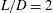$L/D=2$, as a function of the Reynolds number,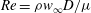$Re=\rho w_{\infty } D /\mu$, and of the rotation parameter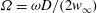$\varOmega =\omega D/(2 w_{\infty })$, in the ranges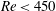$Re<450$ and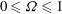$0\leq \varOmega \leq 1$. Here,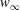$w_{\infty }$ and$\omega$ are the free-stream and the body rotation velocities respectively, and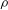$\rho$ and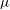$\mu$ are the fluid density and viscosity. The two-dimensional eigenvalue problem (EVP) is solved numerically to find the spectrum of complex eigenvalues and their associated eigenfunctions, allowing us to explain the different bifurcations from the axisymmetric state observed in previous numerical studies. Our results reveal that, for the parameter ranges investigated herein, three global eigenmodes, denoted low-frequency (LF), medium-frequency (MF) and high-frequency (HF) modes, become unstable in different regions of the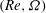$(Re,\varOmega )$-parameter plane. We provide precise computations of the corresponding neutral curves, that divide the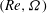$(Re,\varOmega )$-plane into four different regions: the stable axisymmetric flow prevails for small enough values of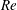$Re$ and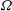$\varOmega$, while three different frozen states, where the wake structures co-rotate with the body at different angular velocities, take place as a consequence of the destabilization of the LF, MF and HF modes. Several direct numerical simulations (DNS) of the nonlinear state associated with the MF mode, identified here for the first time, are also reported to complement the linear stability results. Finally, we point out the important fact that, since the axisymmetric base flow is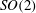$SO(2)$-symmetric, the theory of equivariant bifurcations implies that the weakly nonlinear regimes that emerge close to criticality must necessarily take the form of rotating-wave states. These states, previously referred to as frozen wakes in the literature, are thus shown to result from the base-flow symmetry.

## JFM classification

Type
Papers
Information
Journal of Fluid Mechanics , 10 June 2014 , pp. 302 - 327

## Access options

Get access to the full version of this content by using one of the access options below. (Log in options will check for institutional or personal access. Content may require purchase if you do not have access.)

## References

Achenbach, E. 1974 Vortex shedding from spheres. J. Fluid Mech. 62, 209221.CrossRefGoogle Scholar
Arnoldi, W. E. 1951 The principle of minimized iterations in the solution of the matrix eigenvalue problem. Q. Appl. Maths 9, 1729.CrossRefGoogle Scholar
Blackburn, H. M. & Lopez, J. M. 2002 Modulated rotating waves in an enclosed swirling flow. J. Fluid Mech. 465, 3358.CrossRefGoogle Scholar
Bohorquez, P., Sanmiguel-Rojas, E., Sevilla, A., Jiménez-González, J. I. & Martínez-Bazán, C. 2011 Stability and dynamics of the laminar wake past a slender blunt-based axisymmetric body. J. Fluid Mech. 676, 110144.CrossRefGoogle Scholar
Bouchet, G., Mebarek, M. & Dušek, J. 2006 Hydrodynamics forces acting on a rigid fixed sphere in early transitional regimes. Eur. J. Mech. (B/Fluids) 25, 321336.CrossRefGoogle Scholar
Chomaz, J.-M. 2005 Global instabilities in spatially developing flows: non-normality and nonlinearity. Annu. Rev. Fluid Mech. 37, 357392.CrossRefGoogle Scholar
Crawford, J. D. & Knobloch, E. 1991 Symmetry and symmetry-breaking bifurcations in fluid dynamics. Annu. Rev. Fluid Mech. 23, 341387.CrossRefGoogle Scholar
Fabre, D., Auguste, F. & Magnaudet, J. 2008 Bifurcations and symmetry breaking in the wake of axisymmetric bodies. Phys. Fluids 20, 051702.CrossRefGoogle Scholar
Ferziger, J. H. & Perić, M. 2002 Computational Methods for Fluid Dynamics. Springer.CrossRefGoogle Scholar
Gallaire, F., Ruith, M., Meiburg, E., Chomaz, J.-M. & Huerre, P. 2006 Spiral vortex breakdown as a global mode. J. Fluid Mech. 549, 7180.CrossRefGoogle Scholar
Gerritsma, M. I. & Phillips, T. N. 2000 Spectral element methods for axisymmetric Stokes problems. J. Comput. Phys. 164, 81103.CrossRefGoogle Scholar
Ghidersa, B. & Dušek, J. 2000 Breaking of axisymmetry and onset of unsteadiness in the wake of a sphere. J. Fluid Mech. 423, 3369.CrossRefGoogle Scholar
Golubitsky, M., LeBlanc, V. G. & Melbourne, I. 2000 Hopf birfurcation from rotating waves and patterns in physiscal space. J. Nonlinear Sci. 10, 69101.CrossRefGoogle Scholar
Jasak, H.1996 Error analysis and estimation in the finite volume method with applications to fluid flows. PhD thesis, Imperial College, University of Princeton.Google Scholar
Jiménez-González, J. I., Sanmiguel-Rojas, E., Sevilla, A. & Martínez-Bazán, C. 2013 Laminar flow past a spinning bullet-shaped body at moderate angular velocities. J. Fluids Struct. 43, 200219.CrossRefGoogle Scholar
Johnson, T. A. & Patel, V. C. 1999 Flow past a sphere up to Reynolds number of 300. J. Fluid Mech. 378, 1970.CrossRefGoogle Scholar
Khorrami, M. R. 1991 On the viscous modes of instability of a trailing line vortex. J. Fluid Mech. 225, 197212.CrossRefGoogle Scholar
Khorrami, M. R., Malik, M. R. & Ash, R. L. 1989 Application of spectral collocation techniques to the stability of swirling flows. J. Comput. Phys. 81, 206229.CrossRefGoogle Scholar
Kim, D. & Choi, H. 2002 Laminar flow past a sphere rotating in the streamwise direction. J. Fluid Mech. 461, 365386.CrossRefGoogle Scholar
Langford, W. F., Tagg, R., Kostelich, E. J., Swinney, H. L. & Golubitsky, M. 1988 Primary instabilities and bicriticallity in flow between counter-rotating cylinder. Phys. Fluids 31 (4), 776785.CrossRefGoogle Scholar
Lehoucq, R., Sorensen, D. & Yang, C. 1998 ARPACK Users’ Guide. Solution of Large-Scale Eigenvalue Problems with Implicity Restarted Arnoldi Methods. SIAM.CrossRefGoogle Scholar
Lele, S. K. 1992 Compact finite difference schemes with spectral-like resolution. J. Comput. Phys. 103, 1642.CrossRefGoogle Scholar
Lopez, J. M., Marques, F. & Sanchez, J. 2001 Oscillatory modes in an enclosed swirling flow. J. Fluid Mech. 439, 109129.CrossRefGoogle Scholar
Meliga, P., Chomaz, J.-M. & Sipp, D. 2009 Unsteadiness in the wake of disks and spheres: instability, receptivity and control using direct and adjoint global stability analyses. J. Fluids Struct. 25, 601616.CrossRefGoogle Scholar
Moin, P. 2010 Fundamentals of Engineering Numerical Analysis. Cambridge University Press.CrossRefGoogle Scholar
Monkewitz, P. A. 1988a The absolute and convective nature of instability in two-dimensional wakes at low Reynolds numbers. Phys. Fluids 31, 9991006.CrossRefGoogle Scholar
Monkewitz, P. A. 1988b A note on vortex shedding from axisymmetric bluff bodies. J. Fluid Mech. 192, 561575.CrossRefGoogle Scholar
Natarajan, R. & Acrivos, A. 1993 The instability of the steady flow past spheres and disks. J. Fluid Mech. 254, 323344.CrossRefGoogle Scholar
Oberleithner, K., Sieber, M., Nayeri, C. N., Paschereit, C. O., Petz, C., Hege, H.-C., Noak, B. R. & Wygnanski, I. 2011 Three-dimensional coherent structures in a swirling jet undergoing vortex breakdown: stability analysis and empirical mode construction. J. Fluid Mech. 679, 383414.CrossRefGoogle Scholar
Pier, B. 2008 Local and global instabilities in the wake of a sphere. J. Fluid Mech. 603, 3961.CrossRefGoogle Scholar
Pier, B. 2013 Periodic and quasiperiodic vortex shedding in the wake of a rotating sphere. J. Fluids Struct. 41, 4350.CrossRefGoogle Scholar
Richardson, L. F. 1910 The approximate arithmetical solution by finite diferences of physical problems involving differential equations with an application to the stresses in a masonry dam. Philos. T. R. Soc. A 210, 307357.CrossRefGoogle Scholar
Roache, P. J. 1994 Perspective: a method for uniform reporting of grid refinement studies. J. Fluids Eng. 116 (3), 405413.CrossRefGoogle Scholar
Ruelle, D. 1973 Bifurcations in the presence of a symmetry group. Arch. Rat. Mech. Anal. 51, 136152.CrossRefGoogle Scholar
Sanmiguel-Rojas, E., Jiménez-González, J. I., Bohorquez, P., Pawlak, G. & Martínez-Bazán, C. 2011 Effect of base cavities on the stability of the wake behind slender blunt-based axisymmetric bodies. Phys. Fluids 23, 114103.CrossRefGoogle Scholar
Sanmiguel-Rojas, E., Sevilla, A., Martínez-Bazán, C. & Chomaz, J.-M. 2009 Global mode analysis of axisymmetric bluff-body wakes: stabilization by base bleed. Phys. Fluids 21, 114102.CrossRefGoogle Scholar
Sevilla, A. & Martínez-Bazán, C. 2004 Vortex shedding in high Reynolds number axisymmetric bluff-body wakes: local linear instability and global bleed control. Phys. Fluids 16 (9), 34603469.CrossRefGoogle Scholar
Sipp, D., Marquet, O., Meliga, P. & Barbagallo, A. 2010 Dynamics and control of global instabilities in open-flows: a linearized approach. Appl. Mech. Rev. 63, 030801.CrossRefGoogle Scholar
Tchoufag, J., Magnaudet, J. & Fabre, D. 2013 Linear stability and sensitivity of the flow past a fixed oblate spheroidal bubble. Phys. Fluids 25, 054108.CrossRefGoogle Scholar
Theofilis, V. 2003 Advances in global linear instability analysis of nonparallel and three-dimensional flows. Prog. Aerosp. Sci. 39, 249315.CrossRefGoogle Scholar
Theofilis, V. 2011 Global linear instability. Annu. Rev. Fluid Mech. 43, 319352.CrossRefGoogle Scholar
Tomboulides, A. & Orszag, S. 2000 Numerical investigation of transitional and weak turbulent flow past a sphere. J. Fluid Mech. 416, 4573.CrossRefGoogle Scholar